# Introduction

The funmediation package fits a functional mediation model to a dataset consisting of intensive longitudinal data for a sample of individuals. For each individual $$i$$, the model assumes a time-invariant (i.e., non-time-varying) randomized treatment or exposure $$X_i$$, a distal outcome $$Y_i$$ observed at one time point (e.g., end-of-study), and a time-varying mediator $$M_i(t)$$ observed repeatedly during the interval after $$X_i$$ is observed and before $$Y_i$$ is observed. $$X_i$$ is treated as a number; that means it can either be dichotomous (and coded as 0 for no and 1 for yes) or continuous (and entered as the number). If $$X_i$$ is categorical with more than two categories, it needs to be entered as multiple binary dummy codes. The research question is whether the effect of $$X_i$$ on $$Y_i$$ is mediated by the process $$M_i(t)$$. A similar model was first proposed by Lindquist (2012). $$M_i(t)$$ can be continuous or binary, and $$Y_i$$ can also be either continuous or binary. The treatment variable $$X_i$$ is assumed to be binary.

# Notation

The mediation model for funmediation is fit in stages.

First, the effect of $$X_i$$ on $$M_i$$ is fit behind the scenes as a time-varying effects (longitudinal varying coefficients) model (TVEM; see Hastie and Tibshirani, 1993; Tan et al., 2012). The assumed mean model is $$E(M_i(t)) = \alpha_0(t) + \alpha_X(t) X_i$$ for continuous $$M_i(t)$$, or $$\mathrm{logit}^{-1}(E(M_i(t))) = \alpha_0(t) + \alpha_X(t) X_i$$ for binary $$M_i(t)$$. This fits the marginal (population-averaged) mean: that is, without random effects but with a sandwich covariance estimate to handle within-subject correlation, as in working-independence generalized estimating equations.
This part of the model is fit using the tvem function in the tvem R package.

Next, the effect of $$X_i$$ and $$M_i(t)$$ on $$Y_i$$ is modeled as a scalar-on-function functional regression (see Goldsmith et al., 2011). The assumed mean model is either $$E(Y_i) = \beta_0 + \beta_{X}X_i+ \int\beta_M(t)M_i(t)dt$$ for continuous $$Y_i$$, or $$\mathrm{logit}^{-1}(E(Y_i)) = \beta_0 + \beta_{X}X_i+ \int\beta_M(t)M_i(t)dt$$ for binary $$Y_i$$.
This part of the model is fit using the pfr function in the refund R package (see Goldsmith et al., 2011).
Both the tvem and refund packages use the mgcv package (see Wood, 2017) for back-end calculations.

In this mediation model, $$\alpha_X(t)$$ and $$\beta_M(t)$$ both must be nonzero in order for an indirect effect (i.e., mediation) to exist. These two functions arise conceptually from two different kinds of functional regression; one represents a model with a concurrent function as a response (of which TVEM is a special case), while the other represents a model with a distal scalar response (see Ramsay and Silverman, 2005). However, intuitively the size of the indirect effect has to do with both of them combined. Here we operationally define the indirect effect as the integral $$\int\alpha_X(t)\beta_M(t)dt$$. Because these functions are actually only estimated on a grid of points, the integral is approximated as a weighted average of the cross-products of the estimates. We obtain a bootstrap confidence interval for this quantity using the boot package.

# Including Covariates

Covariates can be included in predicting $$M_i(t)$$ and $$Y_i$$. For example, suppose there is a covariate $$Z_i$$ which has a time-varying relationship to $$M_i(t)$$. The TVEM model for the mediator can be expanded to $$E(M_i(t)) = \alpha_0(t) + \alpha_X(t) X_i + \alpha_Z(t) Z_i$$ for continuous $$M_i(t)$$, or $$\mathrm{logit}^{-1}(E(M_i(t))) = \alpha_0(t) + \alpha_X(t) X_i+ \alpha_Z(t) Z_i$$ for binary $$M_i(t)$$. It is possible for $$Z_i$$ to have a time-varying effect even if the values of $$Z_i$$ do not vary over time. That would mean that the correlation between the observation-level $$M_i(t)$$ and the subject-level $$Z_i$$ is different for different values of $$t$$. It is currently not possible to use a time-varying covariate in this package.

But it is possible to assume that the relationship between $$Z_i$$ and $$M_i(t)$$ does not depend on $$t$$, whether or not $$Z_i$$ depends on $$t$$; in this case $$\alpha_Z(t)$$ can simply be written as $$\alpha_Z$$. The package allows both time-varying-effects and time-invariant-effects of covariates to be specified in predicting the mediator, using the tve_covariates_on_mediator and tie_covariates_on_mediator arguments, respectively.

Alternatively, suppose that there is a subject-level covariate, $$S_i$$, which predicts $$Y_i$$. This can be added to the functional regression model by specifying $$E(Y_i) = \beta_0 + \beta_{X}X_i+ \beta_S S_i + \int\beta_M(t)M_i(t)dt$$ for continuous $$Y_i$$, or $$\mathrm{logit}^{-1}(E(Y_i)) = \beta_0 + \beta_{X}X_i+ \beta_S S_i + \int\beta_M(t)M_i(t)dt$$ for binary $$Y_i$$. Such a covariate can be included using the covariates_on_outcome. Currently, the package assumes a subject-level $$Y_i$$ and does not support multiple functional coefficients in predicting the outcome; that is, the covariates in covariates_on_outcome cannot have time-varying values or effects.

# Example with Continuous Outcomes

The following example shows how to simulate example data and then analyze it using the funmediation package.

## Getting ready to run the example

Before running the examples, first install and load the funmediation package.
A .zip or .tar.gz file containing the package is available at https://github.com/dziakj1/funmediation_development, and it can then be used with the install.packages() function in R code, Packages > Install Package(s) from Local Files in the R graphical user interface, or Tools > Install Packages in the RStudio application, to install the package.

We plan to release funmediation on the CRAN archive, so that it can be installed using the command

install.packages("funmediation")

Another option is to install the package by code from the GitHub repository as follows:

install.packages("devtools")
library(devtools)
install_github("dziakj1/funmediation")

Of course, if you are viewing this guide from within R using the vignette() function, then the package is already installed.

The next step is to load the required packages.

library(tvem)
#> This is mgcv 1.8-38. For overview type 'help("mgcv-package")'.
library(refund)
library(boot)
#>
#> Attaching package: 'boot'
#> The following object is masked from 'package:refund':
#>
#>     cd4
library(funmediation)

We then set a seed for replicability and simulate data.

set.seed(123)
simulation1 <- simulate_funmediation_example(nsub=500)

The simulation1 object will contain not only the simulated dataset itself, but the true values of the simulated parameters, including the indirect effect.

str(simulation1)
#> List of 8
#>  $time_grid : num [1:100] 0.01 0.02 0.03 0.04 0.05 0.06 0.07 0.08 0.09 0.1 ... #>$ true_alpha_int: num [1:100] 0.1 0.141 0.173 0.2 0.224 ...
#>  $true_alpha_X : num [1:100] -0.0707 -0.1 -0.1225 -0.1414 -0.1581 ... #>$ true_beta_int : num 0
#>  $true_beta_M : num [1:100] 0.00503 0.0101 0.01523 0.02041 0.02564 ... #>$ true_beta_X   : num 0.2
#>  $true_indirect : num -0.211 #>$ dataset       :'data.frame':  20169 obs. of  5 variables:
#>   ..$subject_id: int [1:20169] 1 1 1 1 1 1 1 1 1 1 ... #> ..$ t         : num [1:20169] 0.04 0.07 0.11 0.15 0.18 0.19 0.25 0.28 0.29 0.31 ...
#>   ..$X : int [1:20169] 0 0 0 0 0 0 0 0 0 0 ... #> ..$ M         : num [1:20169] 1.165 -0.653 -1.462 2.287 2.271 ...
#>   ..$Y : num [1:20169] -0.181 -0.181 -0.181 -0.181 -0.181 ... We need the simulated dataset as a data.frame object. the_data <- simulation1$dataset

We can use the head and summary function in R, in order to look at the first few lines of the data and univariate descriptive statistics.

print(head(the_data))
#>   subject_id    t X          M        Y
#> 1          1 0.04 0  1.1647240 -0.18087
#> 2          1 0.07 0 -0.6525125 -0.18087
#> 3          1 0.11 0 -1.4620807 -0.18087
#> 4          1 0.15 0  2.2871677 -0.18087
#> 5          1 0.18 0  2.2713836 -0.18087
#> 6          1 0.19 0  1.4810598 -0.18087
summary(the_data)
#>    subject_id        t                X               M
#>  Min.   :  1   Min.   :0.0100   Min.   :0.000   Min.   :-7.1295
#>  1st Qu.:125   1st Qu.:0.2500   1st Qu.:0.000   1st Qu.:-0.9189
#>  Median :251   Median :0.5000   Median :0.000   Median : 0.4338
#>  Mean   :251   Mean   :0.5041   Mean   :0.472   Mean   : 0.4349
#>  3rd Qu.:376   3rd Qu.:0.7600   3rd Qu.:1.000   3rd Qu.: 1.7870
#>  Max.   :500   Max.   :1.0000   Max.   :1.000   Max.   : 7.7515
#>        Y
#>  Min.   :-2.9565
#>  1st Qu.:-0.4219
#>  Median : 0.2832
#>  Mean   : 0.3322
#>  3rd Qu.: 1.0521
#>  Max.   : 3.7903

It would be reasonable to do other descriptive analyses but in order to demonstrate the function we proceed immediately to the main analysis.

# Running a Functional Mediation Model

Now we call the functional mediation function. Only 10 bootstrap samples are used below for this quick illustration. At least a few hundred bootstraps are recommended in practice in order to increase precision and power.

model1 <- funmediation(data=the_data,
treatment=X,
mediator=M,
outcome=Y,
id=subject_id,
time=t,
nboot=10)
#> Warning in funmediation(data = the_data, treatment = X, mediator = M, outcome =
#> Y, : The number of bootstrap samples was very small and results will be dubious.
#> Warning in norm.inter(t, (1 + c(conf, -conf))/2): extreme order statistics used
#> as endpoints
#> Warning in norm.inter(t, alpha): extreme order statistics used as endpoints
#> Warning in norm.inter(t, (1 + c(conf, -conf))/2): extreme order statistics used
#> as endpoints
#> Warning in norm.inter(t, alpha): extreme order statistics used as endpoints

The warning message, extreme order statistics used as endpoints,’’ occurs because too few bootstrap samples are used for the bootstrap confidence intervals to be valid; however, we ignore this here in order for the vignette example to run quickly.

The print function gives an initial overview of the results.

print(model1)
#> =======================================================
#> Functional Regression Mediation Function Output
#> =======================================================
#> TREATMENT: X
#> MEDIATOR: M (Assumed Continuous)
#> OUTCOME: Y (Assumed Continuous)
#> =======================================================
#> DIRECT EFFECT OF X ...
#>  Direct effect estimate:
#> -0.07192656
#>  Direct effect standard error:
#> 0.1047166
#>  Direct effect bootstrap estimate:
#> -0.1102083
#>  Direct effect bootstrap confidence interval:
#>   ... by normal method:
#>  -0.2939 ,  0.0735
#> =======================================================
#> INDIRECT EFFECT OF X ...
#>  Indirect effect parametric estimate:
#>  -0.2522696
#>  Indirect effect bootstrap estimate:
#>  -0.2460247
#>  Indirect effect bootstrap confidence interval:
#>   ... by normal method:
#>  -0.3517 ,  -0.1404
#> =======================================================
#> Computation time:
#> Time difference of 9.988521 secs
#> =======================================================
#> Time-varying Effects Model Predicting MEDIATOR from TREATMENT:
#> Response variable:   MEDIATOR
#> Response outcome distribution type: gaussian
#> Time interval:   0.01 to 1
#> Number of subjects:  500
#> Effects specified as time-varying:  (Intercept), TREATMENT
#> You can use the plot function to view the plots.
#>
#> Back-end model fitted in mgcv::bam function:
#> Method fREML
#> Formula:
#> MEDIATOR ~ TREATMENT + s(time, bs = "ps", by = NA, pc = 0, k = 7,
#>     fx = FALSE) + s(time, bs = "ps", by = TREATMENT, pc = 0,
#>     m = c(2, 1), k = 7, fx = FALSE)
#> Pseudolikelihood AIC: 84897.63
#> Pseudolikelihood BIC: 84937.51
#>
#> =======================================================
#> Functional Regression Model Predicting OUTCOME from MEDIATOR
#>   and TREATMENT:
#>
#> Family: gaussian
#>
#> Formula:
#> OUTCOME ~ s(x = MEDIATOR.tmat, by = L.MEDIATOR) + TREATMENT
#>
#> Estimated degrees of freedom:
#> 2  total = 4
#>
#> REML score: 742.2459
#> Scalar terms in functional regression model:
#> (Intercept)   TREATMENT
#>  0.16499534 -0.07192656
#> =======================================================
#> Parametric model for Predicting OUTCOME from TREATMENT
#>   ignoring MEDIATOR:
#>
#> Call:
#> glm(formula = glm_formula)
#>
#> Deviance Residuals:
#>     Min       1Q   Median       3Q      Max
#> -3.4298  -0.7068   0.0100   0.7185   3.6232
#>
#> Coefficients:
#>             Estimate Std. Error t value Pr(>|t|)
#> (Intercept)  0.47332    0.06671   7.096 4.45e-12 ***
#> TREATMENT   -0.30617    0.09730  -3.147  0.00175 **
#> ---
#> Signif. codes:  0 '***' 0.001 '**' 0.01 '*' 0.05 '.' 0.1 ' ' 1
#>
#> (Dispersion parameter for gaussian family taken to be 1.179171)
#>
#>     Null deviance: 598.90  on 499  degrees of freedom
#> Residual deviance: 587.23  on 498  degrees of freedom
#> AIC: 1505.3
#>
#> Number of Fisher Scoring iterations: 2
#>
#> =======================================================

The first part of the output above summarizes the estimated indirect effect and its bootstrap confidence interval. The estimate for the indirect effect $$\int \alpha_X(t)\beta_M(t)$$ is given as about -0248. Choosing the wider confidence interval in order to be conservative, a 95% confidence interval extends from about -0.35 to -0.14. (This interval would not actually be reliable in practice because of the low number of bootstrap samples, which could be remedied by choosing a higher nboot). Thus, there appears to be a significant negative indirect effect of $$X$$ on $$Y$$ mediated through $$M(t)$$.

The second part of the printed output summarizes the TVEM used to predict $$M(t)$$ from $$X$$. It only provides summary information on the model that was fit. The time-varying coefficients are not actually printed, because they are functions rather than single numbers. They can be plotted using the plot function, as described later. They are also stored in the model1 output object, as described later.

The third part summarizes the scalar-on-function functional regression model used to predict $$Y$$ from $$X$$ and $$M(t)$$. This model involves scalar (non-time-varying) coefficients for the intercept and the direct effect of $$X$$, and a functional (time-varying) coefficient for the effect of $$M(t)$$. The scalar coefficients are printed, but as before, the functional coefficient needs to be plotted using the plot function.

The fourth section of the printed output simply presents an estimate of the total effect of $$X$$ on $$Y$$ ignoring $$M(t)$$, obtained using the glm function. $$X$$ is shown to have a total negative effect on $$Y$$, with an estimated coefficient of -0.30617 and $$p$$-value of 0.00175. This suggests that the $$X=1$$ group has statistically significantly lower average $$Y$$ than the $$X=0$$ group, irrespective of $$M$$.

The plot function plots the results. Three kinds of plots are available, represented by the options tvem, pfr, and coef. The tvem plot shows the estimates for $$\alpha_0(t)$$ and $$\alpha_1(t)$$.

plot(model1, what_plot="tvem")
#>  1.529902
#>  1.311829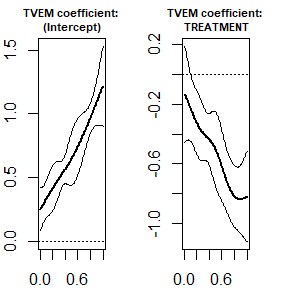The intercept plot appears to be significantly above zero and significantly increasing. It can be interpreted as the mean $$M(t)$$ for the control ($$X=0$$) group because $$E(M(t)|X=0)=\alpha_0(t) + \alpha_X(t) \times 0=\alpha_0(t)$$. The treatment effect plot is generally nonzero and decreasing. It can be interpreted as $$E(M(t)|X=1)-E(M(t)|X=0)$$, a time-specific treatment effect on the mediator, and it suggests that as time goes on the $$X=1$$ group tends to become lower in average $$M(t)$$ than the $$X=0$$ group.

The pfr plot shows the estimate for $$\beta_M(t)$$.

plot(model1, what_plot="pfr")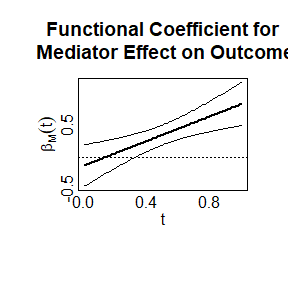The function tends to be nonzero at least for higher values of time, and generally seems to be steadily increasing. One possible interpretation is that the mediator becomes more important at later times. Alternatively, perhaps individuals who increase more (or decrease less) on average over time on the mediator tend to have a higher value on the outcome (see Dziak et al., 2019).

The pfrgam plot does the same thing as the pfr plot, but with a slightly different implementation based on the plot.gam method from the mgcv library.

plot(model1, what_plot="pfrgam")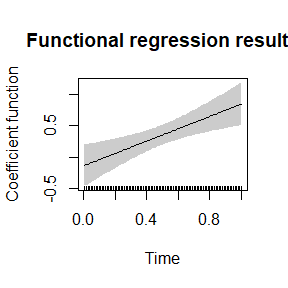Last, the coef plot summarizes all of the most important coefficients estimated in the model. The upper left and upper right panes show the estimates of $$\alpha_0(t)$$ and $$\alpha_X(t)$$ from the model of the effect of $$X$$ on $$M(t)$$. The lower left shows the estimate of $$\beta_M(t)$$ from the model of the effect of $$M(t)$$ on $$Y$$ given $$X$$. In the lower right panel, the estimate of the indirect effect is printed (not plotted, because it is a single number).

plot(model1, what_plot="coef")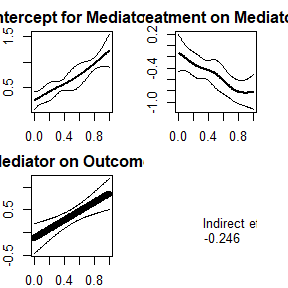Because we have already looked at the relevant plots before using the coef option, there is nothing new to interpret in the coef plot; it is just intended as a convenient summary.

## Exploring Information in the Output Objects

The output object created, called model1, contains useful information both on the model fit to the original data and on the bootstrapping results.

• model1$original_results$time_grid is the grid of time points on which the coefficient functions were estimated.

• model1$original_results$alpha_int_estimate, model1$original_results$alpha_int_se, model1$original_results$alpha_X_estimate, and model1$original_results$alpha_X_se are the functional coefficients and pointwise standard errors for the intercept and treatment in the TVEM, at each point of the time grid.

• model1$original_results$beta_int_estimate, model1$original_results$beta_int_se, model1$original_results$beta_X_estimate and model1$original_results$beta_X_se are the estimates and standard errors for the scalar coefficients representing the intercept and the direct treatment effect in the scalar-outcome functional linear model.

• model1$original_results$beta_M_estimate and model1$original_results$beta_M_se are the estimates and pointwise standard errors representing the effect of the time-varying mediator at each point of the time grid.

• beta_M_pvalue is the $$p$$-value for an overall test of whether the mediator is significantly associated with the outcome, i.e., whether $$\beta_M(t)$$ can be shown not to be all zeroes; see the documentation for the pfr function in the refund package for more information.

• model1$original_results$tau_int_estimate, together with $tau_int_se, $tau_X_estimate, and $tau_X_se, represent the estimates of the intercept and coefficient in the model for the total effect of $$X$$ on $$Y$$. Thus, model1$original_results$tau_X_estimate is the estimated total effect and model1$original_results$tau_X_pvalue is the $$p$$-value for testing whether this effect is zero. • model1$original_results$indirect_effect_estimate gives the estimated value of $$\int \alpha_X(t)\beta_M(t)$$ obtained without bootstrapping. • model1$original_results$tvem_XM_details, $funreg_MY_details, and $total_effect_details give the fitted model objects from tvem, pfr and glm. They might be useful for plots or diagnostics but might contain more technical detail than some users are interested in. ## Including a Covariate It is possible to run the model with one or more additional covariates. To demonstrate this, the simulate_mediation_example has an option make_covariate_S to create an extra subject-level covariate. The data is generated in such a way that $$S$$ is not actually related to $$X$$, $$M$$ or $$Y$$, but the analyst is assumed not to know this – that is, the simulated true value of its coefficient is zero. simulation_with_covariate <- simulate_funmediation_example(nsub=500, make_covariate_S=TRUE); data_with_covariate <- simulation_with_covariate$dataset; 

To include the covariate $$S_i$$ with an assumption of a possibly time-varying effect $$\alpha_S(t)$$ on $$M(t)$$ (recall that the covariate can have a time-varying effect even though it may not have time-varying values), use the covariates_on_outcome and tve_covariates_on_mediator arguments:

model_with_tve_covariate <- funmediation(data=data_with_covariate,
treatment=X,
mediator=M,
outcome=Y,
tve_covariates_on_mediator = ~S,
covariates_on_outcome = ~S,
id=subject_id,
time=t,
nboot=10)
#> Warning in funmediation(data = data_with_covariate, treatment = X, mediator =
#> M, : The number of bootstrap samples was very small and results will be dubious.
#> Warning in norm.inter(t, (1 + c(conf, -conf))/2): extreme order statistics used
#> as endpoints
#> Warning in norm.inter(t, alpha): extreme order statistics used as endpoints
#> Warning in norm.inter(t, (1 + c(conf, -conf))/2): extreme order statistics used
#> as endpoints
#> Warning in norm.inter(t, alpha): extreme order statistics used as endpoints

Notice that the tilde (~) character needs to be included for the covariates. This is done so that R will allow multiple covariates to be listed in the style of the right side of a formula, e.g., ~S1 + S2.

The covariates in tve_covariates_on_mediator and covariates_on_outcome need to be subject-level and should not have time-varying values; the function does not currently support models with time-varying values other than the mediator. However, the relationship of the covariates to the mediator can be specified as either time-varying or time-invariant. The relationship of the covariate to the outcome, in contrast, can only be specified as time- invariant in the current version of the package. That is, $$M(t)$$ is the only time-varying predictor in the functional regression predicting the outcome.

The results of the model fit can be viewed using the print and plot functions:

print(model_with_tve_covariate)
#> =======================================================
#> Functional Regression Mediation Function Output
#> =======================================================
#> TREATMENT: X
#> MEDIATOR: M (Assumed Continuous)
#> OUTCOME: Y (Assumed Continuous)
#> =======================================================
#> DIRECT EFFECT OF X ...
#>  Direct effect estimate:
#> 0.1125763
#>  Direct effect standard error:
#> 0.1009101
#>  Direct effect bootstrap estimate:
#> 0.06677104
#>  Direct effect bootstrap confidence interval:
#>   ... by normal method:
#>  -0.124 ,  0.2575
#> =======================================================
#> INDIRECT EFFECT OF X ...
#>  Indirect effect parametric estimate:
#>  -0.3018684
#>  Indirect effect bootstrap estimate:
#>  -0.2930111
#>  Indirect effect bootstrap confidence interval:
#>   ... by normal method:
#>  -0.3663 ,  -0.2197
#> =======================================================
#> Computation time:
#> Time difference of 19.11419 secs
#> =======================================================
#> Time-varying Effects Model Predicting MEDIATOR from TREATMENT:
#> Response variable:   MEDIATOR
#> Response outcome distribution type: gaussian
#> Time interval:   0.01 to 1
#> Number of subjects:  500
#> Effects specified as time-varying:  (Intercept), TREATMENT, S
#> You can use the plot function to view the plots.
#>
#> Back-end model fitted in mgcv::bam function:
#> Method fREML
#> Formula:
#> MEDIATOR ~ TREATMENT + S + s(time, bs = "ps", by = NA, pc = 0,
#>     k = 7, fx = FALSE) + s(time, bs = "ps", by = TREATMENT, pc = 0,
#>     m = c(2, 1), k = 7, fx = FALSE) + s(time, bs = "ps", by = S,
#>     pc = 0, m = c(2, 1), k = 7, fx = FALSE)
#> Pseudolikelihood AIC: 83986.37
#> Pseudolikelihood BIC: 84047.99
#>
#> =======================================================
#> Functional Regression Model Predicting OUTCOME from MEDIATOR
#>   and TREATMENT:
#>
#> Family: gaussian
#>
#> Formula:
#> OUTCOME ~ s(x = MEDIATOR.tmat, by = L.MEDIATOR) + TREATMENT +
#>     S
#>
#> Estimated degrees of freedom:
#> 2  total = 5
#>
#> REML score: 729.0772
#> Scalar terms in functional regression model:
#>  (Intercept)    TREATMENT            S
#> -0.025787118  0.112576286 -0.001643264
#> =======================================================
#> Parametric model for Predicting OUTCOME from TREATMENT
#>   ignoring MEDIATOR:
#>
#> Call:
#> glm(formula = glm_formula)
#>
#> Deviance Residuals:
#>      Min        1Q    Median        3Q       Max
#> -3.02647  -0.72182  -0.00592   0.67548   2.95742
#>
#> Coefficients:
#>             Estimate Std. Error t value Pr(>|t|)
#> (Intercept)  0.36962    0.08331   4.437 1.12e-05 ***
#> TREATMENT   -0.16672    0.09601  -1.737   0.0831 .
#> S           -0.02827    0.09593  -0.295   0.7684
#> ---
#> Signif. codes:  0 '***' 0.001 '**' 0.01 '*' 0.05 '.' 0.1 ' ' 1
#>
#> (Dispersion parameter for gaussian family taken to be 1.149205)
#>
#>     Null deviance: 574.74  on 499  degrees of freedom
#> Residual deviance: 571.16  on 497  degrees of freedom
#> AIC: 1493.5
#>
#> Number of Fisher Scoring iterations: 2
#>
#> =======================================================
plot(model_with_tve_covariate, what_plot="tvem")
#>  1.49397
#>  1.138447
#>  0.8460518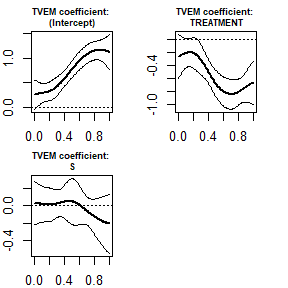Notice that we now get a time-varying effects plot which includes a coefficient for the effect of $$S$$, in addition to the coefficients for the effect of $$X$$. In this example $$\alpha_S(t)$$ is not significantly nonzero at any time and does not change over time, so there is no evidence that covariate $$S$$ is important in predicting the outcome $$Y$$. As before, the $$X=1$$ group has lower values on the mediator than the $$X=0$$ group (i.e., their contrast is negative) at least at later time points.

Alternatively, by specifying tie_covariates_on_mediator instead of tve_covariates_on_mediator, the covariate can be assumed to have a time-invariant effect rather than a time-varying effect on $$M$$.

model_with_tie_covariate <- funmediation(data=data_with_covariate,
treatment=X,
mediator=M,
outcome=Y,
tie_covariates_on_mediator = ~S,
covariates_on_outcome = ~S,
id=subject_id,
time=t,
nboot=10)
#> Warning in funmediation(data = data_with_covariate, treatment = X, mediator =
#> M, : The number of bootstrap samples was very small and results will be dubious.
#> Warning in norm.inter(t, (1 + c(conf, -conf))/2): extreme order statistics used
#> as endpoints
#> Warning in norm.inter(t, alpha): extreme order statistics used as endpoints
#> Warning in norm.inter(t, (1 + c(conf, -conf))/2): extreme order statistics used
#> as endpoints
#> Warning in norm.inter(t, alpha): extreme order statistics used as endpoints
print(model_with_tie_covariate)
#> =======================================================
#> Functional Regression Mediation Function Output
#> =======================================================
#> TREATMENT: X
#> MEDIATOR: M (Assumed Continuous)
#> OUTCOME: Y (Assumed Continuous)
#> =======================================================
#> DIRECT EFFECT OF X ...
#>  Direct effect estimate:
#> 0.1125763
#>  Direct effect standard error:
#> 0.1009101
#>  Direct effect bootstrap estimate:
#> 0.11074
#>  Direct effect bootstrap confidence interval:
#>   ... by normal method:
#>  -0.0443 ,  0.2658
#> =======================================================
#> INDIRECT EFFECT OF X ...
#>  Indirect effect parametric estimate:
#>  -0.302025
#>  Indirect effect bootstrap estimate:
#>  -0.2680548
#>  Indirect effect bootstrap confidence interval:
#>   ... by normal method:
#>  -0.3417 ,  -0.1944
#> =======================================================
#> Computation time:
#> Time difference of 13.96855 secs
#> =======================================================
#> Time-varying Effects Model Predicting MEDIATOR from TREATMENT:
#> Response variable:   MEDIATOR
#> Response outcome distribution type: gaussian
#> Time interval:   0.01 to 1
#> Number of subjects:  500
#> Effects specified as time-varying:  (Intercept), TREATMENT
#> You can use the plot function to view the plots.
#>
#> Effects specified as non-time-varying:
#>      estimate standard_error
#> S -0.03008131     0.05790221
#>
#> Back-end model fitted in mgcv::bam function:
#> Method fREML
#> Formula:
#> MEDIATOR ~ TREATMENT + S + s(time, bs = "ps", by = NA, pc = 0,
#>     k = 7, fx = FALSE) + s(time, bs = "ps", by = TREATMENT, pc = 0,
#>     m = c(2, 1), k = 7, fx = FALSE)
#> Pseudolikelihood AIC: 83993.2
#> Pseudolikelihood BIC: 84042.46
#>
#> =======================================================
#> Functional Regression Model Predicting OUTCOME from MEDIATOR
#>   and TREATMENT:
#>
#> Family: gaussian
#>
#> Formula:
#> OUTCOME ~ s(x = MEDIATOR.tmat, by = L.MEDIATOR) + TREATMENT +
#>     S
#>
#> Estimated degrees of freedom:
#> 2  total = 5
#>
#> REML score: 729.0772
#> Scalar terms in functional regression model:
#>  (Intercept)    TREATMENT            S
#> -0.025787118  0.112576286 -0.001643264
#> =======================================================
#> Parametric model for Predicting OUTCOME from TREATMENT
#>   ignoring MEDIATOR:
#>
#> Call:
#> glm(formula = glm_formula)
#>
#> Deviance Residuals:
#>      Min        1Q    Median        3Q       Max
#> -3.02647  -0.72182  -0.00592   0.67548   2.95742
#>
#> Coefficients:
#>             Estimate Std. Error t value Pr(>|t|)
#> (Intercept)  0.36962    0.08331   4.437 1.12e-05 ***
#> TREATMENT   -0.16672    0.09601  -1.737   0.0831 .
#> S           -0.02827    0.09593  -0.295   0.7684
#> ---
#> Signif. codes:  0 '***' 0.001 '**' 0.01 '*' 0.05 '.' 0.1 ' ' 1
#>
#> (Dispersion parameter for gaussian family taken to be 1.149205)
#>
#>     Null deviance: 574.74  on 499  degrees of freedom
#> Residual deviance: 571.16  on 497  degrees of freedom
#> AIC: 1493.5
#>
#> Number of Fisher Scoring iterations: 2
#>
#> =======================================================
plot(model_with_tie_covariate, what_plot="pfr")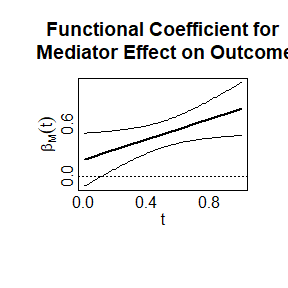The functional effect plot here is very similar to what it was before. Now there are scalar estimates for $$\alpha_S=-0.03008$$ and $$\beta_S=-0.00164$$ but no plot for $$\alpha_S(t)$$ because $$\alpha_S$$ is now assumed not to vary over time. Again, $$S$$ is not statistically significant either as a predictor of the mediator or of the outcome, because the coefficients are not large relative to their standard errors.

## Using a Binary Mediator

The mediator may be binary rather than continuous, in which case the the TVEM uses the logistic link function. For purposes of this vignette demonstration, we dichotomize $$M$$ in the simulated dataset and use that as our binary mediator (this usually would not be advisable for real data because it involves loss of information).

data_with_covariate$binary_M <- 1*(data_with_covariate$M > mean(data_with_covariate$M)) head(data_with_covariate) #> subject_id t X M Y S binary_M #> 1 1 0.01 1 -2.9541303 -0.44206 0 0 #> 2 1 0.05 1 -0.5834873 -0.44206 0 0 #> 3 1 0.06 1 0.6919501 -0.44206 0 1 #> 4 1 0.08 1 0.4411048 -0.44206 0 1 #> 5 1 0.15 1 -1.2007641 -0.44206 0 0 #> 6 1 0.17 1 -1.0369571 -0.44206 0 0 Now we call the funmediation function and include the binary_mediator=TRUE argument. model_with_binary_M <- funmediation(data=data_with_covariate, treatment=X, mediator=binary_M, outcome=Y, id=subject_id, time=t, binary_mediator=TRUE, nboot=10) #> Warning in funmediation(data = data_with_covariate, treatment = X, mediator = #> binary_M, : The number of bootstrap samples was very small and results will be #> dubious. #> Warning in norm.inter(t, (1 + c(conf, -conf))/2): extreme order statistics used #> as endpoints #> Warning in norm.inter(t, alpha): extreme order statistics used as endpoints #> Warning in norm.inter(t, (1 + c(conf, -conf))/2): extreme order statistics used #> as endpoints #> Warning in norm.inter(t, alpha): extreme order statistics used as endpoints print(model_with_binary_M) #> ======================================================= #> Functional Regression Mediation Function Output #> ======================================================= #> TREATMENT: X #> MEDIATOR: binary_M (Assumed Binary) #> OUTCOME: Y (Assumed Continuous) #> ======================================================= #> DIRECT EFFECT OF X ... #> Direct effect estimate: #> 0.03910025 #> Direct effect standard error: #> 0.1004427 #> Direct effect bootstrap estimate: #> 0.07949018 #> Direct effect bootstrap confidence interval: #> ... by normal method: #> -0.1906 , 0.3497 #> ======================================================= #> INDIRECT EFFECT OF X ... #> Indirect effect parametric estimate: #> -0.8550336 #> Indirect effect bootstrap estimate: #> -0.2207669 #> Indirect effect bootstrap confidence interval: #> ... by normal method: #> -0.3184 , -0.1231 #> ======================================================= #> Computation time: #> Time difference of 17.59376 secs #> ======================================================= #> Time-varying Effects Model Predicting MEDIATOR from TREATMENT: #> Response variable: MEDIATOR #> Response outcome distribution type: binomial #> Time interval: 0.01 to 1 #> Number of subjects: 500 #> Effects specified as time-varying: (Intercept), TREATMENT #> You can use the plot function to view the plots. #> #> Back-end model fitted in mgcv::bam function: #> Method fREML #> Formula: #> MEDIATOR ~ TREATMENT + s(time, bs = "ps", by = NA, pc = 0, k = 7, #> fx = FALSE) + s(time, bs = "ps", by = TREATMENT, pc = 0, #> m = c(2, 1), k = 7, fx = FALSE) #> Pseudolikelihood AIC: 27100.7 #> Pseudolikelihood BIC: 27134.79 #> #> ======================================================= #> Functional Regression Model Predicting OUTCOME from MEDIATOR #> and TREATMENT: #> #> Family: gaussian #> Link function: identity #> #> Formula: #> OUTCOME ~ s(x = MEDIATOR.tmat, by = L.MEDIATOR) + TREATMENT #> #> Estimated degrees of freedom: #> 2 total = 4 #> #> REML score: 731.6047 #> Scalar terms in functional regression model: #> (Intercept) TREATMENT #> -0.62912300 0.03910025 #> ======================================================= #> Parametric model for Predicting OUTCOME from TREATMENT #> ignoring MEDIATOR: #> #> Call: #> glm(formula = glm_formula) #> #> Deviance Residuals: #> Min 1Q Median 3Q Max #> -3.01293 -0.71639 -0.01174 0.68195 2.97096 #> #> Coefficients: #> Estimate Std. Error t value Pr(>|t|) #> (Intercept) 0.35608 0.06942 5.129 4.18e-07 *** #> TREATMENT -0.16720 0.09591 -1.743 0.0819 . #> --- #> Signif. codes: 0 '***' 0.001 '**' 0.01 '*' 0.05 '.' 0.1 ' ' 1 #> #> (Dispersion parameter for gaussian family taken to be 1.147098) #> #> Null deviance: 574.74 on 499 degrees of freedom #> Residual deviance: 571.25 on 498 degrees of freedom #> AIC: 1491.6 #> #> Number of Fisher Scoring iterations: 2 #> #> ======================================================= plot(model_with_binary_M, what_plot="coef")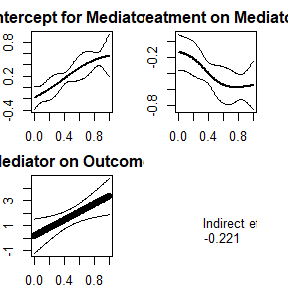The plots are similar to those shown in the previous example with numerical mediator, except that the TVEM coefficient functions for the intercept and treatment effects on the outcome are interpreted on the logit scale as in logistic regression. Note that if the binary_mediator=TRUE argument is not specified, the TVEM will be fit using an identity link (hence a linear model at any given time $$t$$) as for a normally distributed outcome. The function would not detect that binary_M consists of zeroes and ones and no other numbers. The identity link is probably not the best approach but might be interesting as a comparison. If the mediator is binary, it is probably better to use binary_mediator=TRUE in order to specify that the $$\alpha$$ coefficients are to be interpreted on the logit (logistic regression) scale. A future version of this package might allow log link functions as an alternative to logit link functions, but this is currently not available. # Example with a Binary Outcome In addition to, or instead of, a binary mediator, the outcome may also binary. In this case, the scalar-on-function part of the model (the $$\beta_0$$, $$\beta_X$$ and $$\beta_M(t)$$ functions) are fit using a logistic link function. To demonstrate this, we first simulate a dataset with a binary outcome, using the simulate_binary_Y argument in simulate_funmediation_example function. We set this argument to TRUE, as it is FALSE by default. (Note that we do not currently have a corresponding simulate_binary_M argument in this function, as simulating realistic binary data trajectories is somewhat more difficult.) simulation_with_binary_Y <- simulate_funmediation_example(simulate_binary_Y=TRUE, nsub=500) data_with_binary_Y <- simulation_with_binary_Y$dataset
#>   subject_id    t X           M Y
#> 1          1 0.01 1  0.58107828 0
#> 2          1 0.02 1  3.99963771 0
#> 3          1 0.03 1  1.54573431 0
#> 4          1 0.08 1  1.37180632 0
#> 5          1 0.09 1 -0.05694934 0
#> 6          1 0.15 1 -2.15847027 0

Now we fit the model, using the new dataset with binary $$Y$$, and using the binary_outcome=TRUE argument. By default, binary_outcome is FALSE. The binary_outcome=TRUE argument specifies that the $$\beta$$ coefficients are to be interpreted as logistic regression coefficients, similar to the binary_mediator=TRUE argument which does the same for the $$\alpha$$ coefficients.

model_with_binary_Y <- funmediation(data=data_with_binary_Y,
treatment=X,
mediator=M,
outcome=Y,
id=subject_id,
time=t,
binary_outcome=TRUE,
nboot=10)
#> Warning in funmediation(data = data_with_binary_Y, treatment = X, mediator =
#> M, : The number of bootstrap samples was very small and results will be dubious.
#> Warning in norm.inter(t, (1 + c(conf, -conf))/2): extreme order statistics used
#> as endpoints
#> Warning in norm.inter(t, alpha): extreme order statistics used as endpoints
#> Warning in norm.inter(t, (1 + c(conf, -conf))/2): extreme order statistics used
#> as endpoints
#> Warning in norm.inter(t, alpha): extreme order statistics used as endpoints
print(model_with_binary_Y)
#> =======================================================
#> Functional Regression Mediation Function Output
#> =======================================================
#> TREATMENT: X
#> MEDIATOR: M (Assumed Continuous)
#> OUTCOME: Y (Assumed Binary)
#> =======================================================
#> DIRECT EFFECT OF X ...
#>  Direct effect estimate:
#> -0.02380259
#>  Direct effect standard error:
#> 0.2002658
#>  Direct effect bootstrap estimate:
#> 0.04756748
#>  Direct effect bootstrap confidence interval:
#>   ... by normal method:
#>  -0.4885 ,  0.5837
#> =======================================================
#> INDIRECT EFFECT OF X ...
#>  Indirect effect parametric estimate:
#>  -0.1556537
#>  Indirect effect bootstrap estimate:
#>  -0.1477143
#>  Indirect effect bootstrap confidence interval:
#>   ... by normal method:
#>  -0.2873 ,  -0.0081
#> =======================================================
#> Computation time:
#> Time difference of 9.535622 secs
#> =======================================================
#> Time-varying Effects Model Predicting MEDIATOR from TREATMENT:
#> Response variable:   MEDIATOR
#> Response outcome distribution type: gaussian
#> Time interval:   0.01 to 1
#> Number of subjects:  500
#> Effects specified as time-varying:  (Intercept), TREATMENT
#> You can use the plot function to view the plots.
#>
#> Back-end model fitted in mgcv::bam function:
#> Method fREML
#> Formula:
#> MEDIATOR ~ TREATMENT + s(time, bs = "ps", by = NA, pc = 0, k = 7,
#>     fx = FALSE) + s(time, bs = "ps", by = TREATMENT, pc = 0,
#>     m = c(2, 1), k = 7, fx = FALSE)
#> Pseudolikelihood AIC: 85466.42
#> Pseudolikelihood BIC: 85505.49
#>
#> =======================================================
#> Functional Regression Model Predicting OUTCOME from MEDIATOR
#>   and TREATMENT:
#>
#> Family: binomial
#>
#> Formula:
#> OUTCOME ~ s(x = MEDIATOR.tmat, by = L.MEDIATOR) + TREATMENT
#>
#> Estimated degrees of freedom:
#> 2  total = 4
#>
#> REML score: 339.0038
#> Scalar terms in functional regression model:
#> (Intercept)   TREATMENT
#>  0.27297288 -0.02380259
#> =======================================================
#> Parametric model for Predicting OUTCOME from TREATMENT
#>   ignoring MEDIATOR:
#>
#> Call:
#> glm(formula = glm_formula, family = binomial)
#>
#> Deviance Residuals:
#>     Min       1Q   Median       3Q      Max
#> -1.3893  -1.3103   0.9794   1.0500   1.0500
#>
#> Coefficients:
#>             Estimate Std. Error z value Pr(>|z|)
#> (Intercept)   0.4855     0.1355   3.583 0.000339 ***
#> TREATMENT    -0.1783     0.1832  -0.973 0.330608
#> ---
#> Signif. codes:  0 '***' 0.001 '**' 0.01 '*' 0.05 '.' 0.1 ' ' 1
#>
#> (Dispersion parameter for binomial family taken to be 1)
#>
#>     Null deviance: 674.60  on 499  degrees of freedom
#> Residual deviance: 673.65  on 498  degrees of freedom
#> AIC: 677.65
#>
#> Number of Fisher Scoring iterations: 4
#>
#> =======================================================
plot(model_with_binary_Y, what_plot="coef")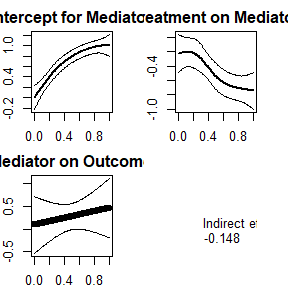Note that the relationship of the mediator function to the outcome is now interpreted as a functional logistic regression coefficient rather than a functional linear regression coefficient (see Dziak et al., 2019; Goldsmith, Crainiceanu, Caffo & Rice, 2011; Mousavi and Srensen, 2018 for descriptions of functional logistic regression).

# More than Two Treatment Groups

In the preceding examples, the treatment was assumed to be dichotomous and dummy-coded (0 for a control group and 1 for a treated group).
Thus, the coefficients were interpreted as effects of increasing the binary variable $$X_i$$ from 0 to 1. However, sometimes in practice there might be more than two groups in the experiment; for example, there might be two different interventions each being compared to the same control group. In this case, $$X_i$$ should not be coded as, say, 1, 2, and 3, for the different groups, because in that case the funmediation function would misinterpret the levels as if they were actually numbers.
To handle this, dummy-code multiple predictor variables so that, e.g., X1 is 1 for treatment group 1 and 0 otherwise, and X2 is 1 for treatment group 2 and 0 otherwise. In this case, the effect of X1 represents the contrast between group 1 and the remaining (reference) group 3, and the effect of X2 represents the contrast between group 2 and the reference group. You could optionally choose a different reference group (e.g., have a dummy code for group 2 and a dummy code for group 3, leaving 1 as the reference level, instead of a dummy code for groups 1 and 2). Except when using data simulated by the package, you should code the predictor variables yourself, keeping in mind which contrasts are most interesting to you. Regardless, one of the treatment levels, perhaps representing the control or reference group, does not get its own predictor variable but is represented indirectly by having zeroes on all the others.

The following code will simulate data from a study with three treatment groups, hence two binary treatment variables.

set.seed(12345)
nboot <- 99
f1 <- function(t) {return(-(t/2)^.5)}
f2 <- function(t) {return(sqrt(t))}
f3 <- function(t) {return(sin(2*3.141593*t))}
the_simulation <- simulate_funmediation_example(nlevels=3,
alpha_X = list(f1,f2),
beta_M = f3,
beta_X = c(.2,.3))

In the code above, notice that alpha_X, the time-varying effect of treatment on the mediator, has to be specified as a list of two functions, corresponding to the two dummy-coded dimensions of treatment. Similarly, beta_X, the direct effect of treatment on outcome, is a vector of two numbers.

The first few lines of the resulting dataset look like this:

head(the_simulation$dataset) #> subject_id t X1 X2 M Y #> 1 1 0.01 1 0 -1.25501308 -2.56836 #> 2 1 0.02 1 0 -0.12442401 -2.56836 #> 3 1 0.03 1 0 0.02886934 -2.56836 #> 4 1 0.06 1 0 -1.64544032 -2.56836 #> 5 1 0.09 1 0 -3.43521092 -2.56836 #> 6 1 0.12 1 0 -0.24772361 -2.56836 The following code will analyze this data: the_data <- the_simulation$dataset
ans_funmed_3groups <- funmediation(data=the_data,
treatment=~X1+X2,
mediator=M,
outcome=Y,
tvem_num_knots=5,
id=subject_id,
time=t,
nboot=10)
#> Warning in funmediation(data = the_data, treatment = ~X1 + X2, mediator = M, :
#> The number of bootstrap samples was very small and results will be dubious.
#> Warning in norm.inter(t, (1 + c(conf, -conf))/2): extreme order statistics used
#> as endpoints
#> Warning in norm.inter(t, alpha): extreme order statistics used as endpoints
#> Warning in norm.inter(t, (1 + c(conf, -conf))/2): extreme order statistics used
#> as endpoints
#> Warning in norm.inter(t, alpha): extreme order statistics used as endpoints
#> Warning in norm.inter(t, (1 + c(conf, -conf))/2): extreme order statistics used
#> as endpoints
#> Warning in norm.inter(t, alpha): extreme order statistics used as endpoints
#> Warning in norm.inter(t, (1 + c(conf, -conf))/2): extreme order statistics used
#> as endpoints
#> Warning in norm.inter(t, alpha): extreme order statistics used as endpoints

The print function works as before to print the model details.

print(ans_funmed_3groups)
#> =======================================================
#> Functional Regression Mediation Function Output
#> =======================================================
#> TREATMENT: X1 X2
#> MEDIATOR: M (Assumed Continuous)
#> OUTCOME: Y (Assumed Continuous)
#> =======================================================
#> DIRECT EFFECT OF X1 ...
#>  Direct effect estimate:
#> 0.0822701
#>  Direct effect standard error:
#> 0.1162471
#>  Direct effect bootstrap estimate:
#> 0.07430718
#>  Direct effect bootstrap confidence interval:
#>   ... by normal method:
#>  -0.0702 ,  0.2188
#> DIRECT EFFECT OF X2 ...
#>  Direct effect estimate:
#> 0.170827
#>  Direct effect standard error:
#> 0.1134873
#>  Direct effect bootstrap estimate:
#> 0.2420447
#>  Direct effect bootstrap confidence interval:
#>   ... by normal method:
#>  0.0137 ,  0.4705
#> =======================================================
#> INDIRECT EFFECT OF X1 ...
#>  Indirect effect parametric estimate:
#>  0.05440521
#>  Indirect effect bootstrap estimate:
#>  0.1091657
#>  Indirect effect bootstrap confidence interval:
#>   ... by normal method:
#>  -0.0879 ,  0.3063
#> INDIRECT EFFECT OF X2 ...
#>  Indirect effect parametric estimate:
#>  -0.02585488
#>  Indirect effect bootstrap estimate:
#>  -0.08227223
#>  Indirect effect bootstrap confidence interval:
#>   ... by normal method:
#>  -0.2954 ,  0.1309
#> =======================================================
#> Computation time:
#> Time difference of 14.3537 secs
#> =======================================================
#> Time-varying Effects Model Predicting MEDIATOR from TREATMENT:
#> Response variable:   MEDIATOR
#> Response outcome distribution type: gaussian
#> Time interval:   0.01 to 1
#> Number of subjects:  500
#> Effects specified as time-varying:  (Intercept), TREATMENT1, TREATMENT2
#> You can use the plot function to view the plots.
#>
#> Back-end model fitted in mgcv::bam function:
#> Method fREML
#> Formula:
#> MEDIATOR ~ TREATMENT1 + TREATMENT2 + s(time, bs = "ps", by = NA,
#>     pc = 0, k = 9, fx = FALSE) + s(time, bs = "ps", by = TREATMENT1,
#>     pc = 0, m = c(2, 1), k = 9, fx = FALSE) + s(time, bs = "ps",
#>     by = TREATMENT2, pc = 0, m = c(2, 1), k = 9, fx = FALSE)
#> Pseudolikelihood AIC: 84878.53
#> Pseudolikelihood BIC: 84943.49
#>
#> =======================================================
#> Functional Regression Model Predicting OUTCOME from MEDIATOR
#>   and TREATMENT:
#>
#> Family: gaussian
#>
#> Formula:
#> OUTCOME ~ s(x = MEDIATOR.tmat, by = L.MEDIATOR) + TREATMENT1 +
#>     TREATMENT2
#>
#> Estimated degrees of freedom:
#> 5.22  total = 8.22
#>
#> REML score: 706.8747
#> Scalar terms in functional regression model:
#> (Intercept)  TREATMENT1  TREATMENT2
#> -0.03696895  0.08227010  0.17082700
#> =======================================================
#> Parametric model for Predicting OUTCOME from TREATMENT
#>   ignoring MEDIATOR:
#>
#> Call:
#> glm(formula = glm_formula)
#>
#> Deviance Residuals:
#>     Min       1Q   Median       3Q      Max
#> -3.3201  -0.7230  -0.0177   0.7054   3.1962
#>
#> Coefficients:
#>             Estimate Std. Error t value Pr(>|t|)
#> (Intercept)  -0.1050     0.0798  -1.315    0.189
#> TREATMENT1    0.1449     0.1146   1.265    0.207
#> TREATMENT2    0.1070     0.1119   0.956    0.339
#>
#> (Dispersion parameter for gaussian family taken to be 1.069747)
#>
#>     Null deviance: 533.53  on 499  degrees of freedom
#> Residual deviance: 531.66  on 497  degrees of freedom
#> AIC: 1457.6
#>
#> Number of Fisher Scoring iterations: 2
#>
#> =======================================================

The pfr plot is essentially the same as before, since there is only one mediator.

plot(ans_funmed_3groups, use_panes=TRUE, what_plot = "pfr")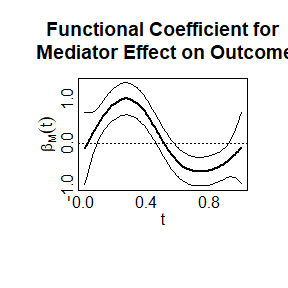In this example, the mediator seems to be positively related to the outcome at earlier times, but weakly negatively related at later times, although substantive interpretation of such functions requires care.

The tvem plot now shows the time-varying effects for both predictors.

plot(ans_funmed_3groups, use_panes=TRUE, what_plot = "tvem")
#>  1.409044
#>  1.447279
#>  1.039191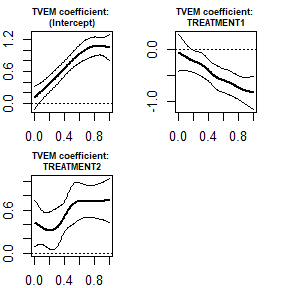The coefficient for the intercept shows that at least for the reference group (group 3 in this example) the expected value of the response tends to increase over time. The coefficient for the first treatment variable (contrasting group 1 vs. group 3) shows a lower value on the mediator for group 1 relative to group 3 (a negative contrast) at least at the later time points. The coefficient for the second treatment variable suggests that group 2 has a higher mean than group 3, especially at later time points.

The coefs plot also includes the extra time-varying effect. The indirect effects are not printed in the lower right pane anymore, because that pane is needed for the new time-varying effect. However, it can be viewed in the output from the print statement as usual.

plot(ans_funmed_3groups, use_panes=TRUE, what_plot = "coefs")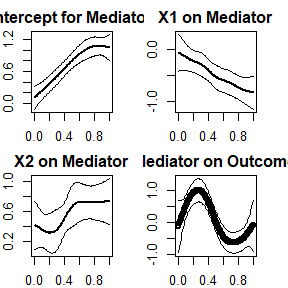Currently the package can still only handle a single mediator variable. It doesn’t currently allow, for example, different mediator variables for different treatment variables.

# Acknowledgements

The pfr function was developed by Jonathan Gellar, Mathew W. McLean, Jeff Goldsmith, and Fabian Scheipl, the boot package was written by Angelo Canty and Brian Ripley, the mgcv package was developed by Simon N. Wood, and the refund package was developed and maintained by Julia Wrobel and others.